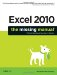L

Index

[SYMBOL] [A] [B] [C] [D] [E] [F] [G] [H] [I] [J] [K] [L] [M] [N] [O] [P] [Q] [R] [S] [T] [U] [V] [W] [X] [Y] [Z]

labels
appearing
axis [See axis labels]
LARGE( ) function
LCM( ) function
Left (Indent) ( cell appearance formatting)
left lookup
LEFT( ) function 2nd
legend (chart)
LEN( ) function
less than (<) operator
less than or equal to (<=) operator
line charts 2nd 3rd
100% stacked
3-D effect
formatting
stacked
with markers
Line Color button
Linear trend
Lines (shape category)
LINEST( ) function
defined
difference between editing embedded objects and
editing
lists
XML mapping
literal values
literals
LN( ) function
loans, calculating
local templates
locked cells
LOG( ) function
LOG10( ) function
logical operators
lookup functions [See HLOOKUP( function; LOOKUP( ) function; VLOOKUP( ) function)]
defined
Lookup Wizard
LOOKUP( ) function
LOOKUP.XLA
lookups, double
loops
LOWER( ) functionExcel 2010: The Missing Manual
ISBN: 1449382355
EAN: 2147483647
Year: 2003
Pages: 185

Similar book on Amazon